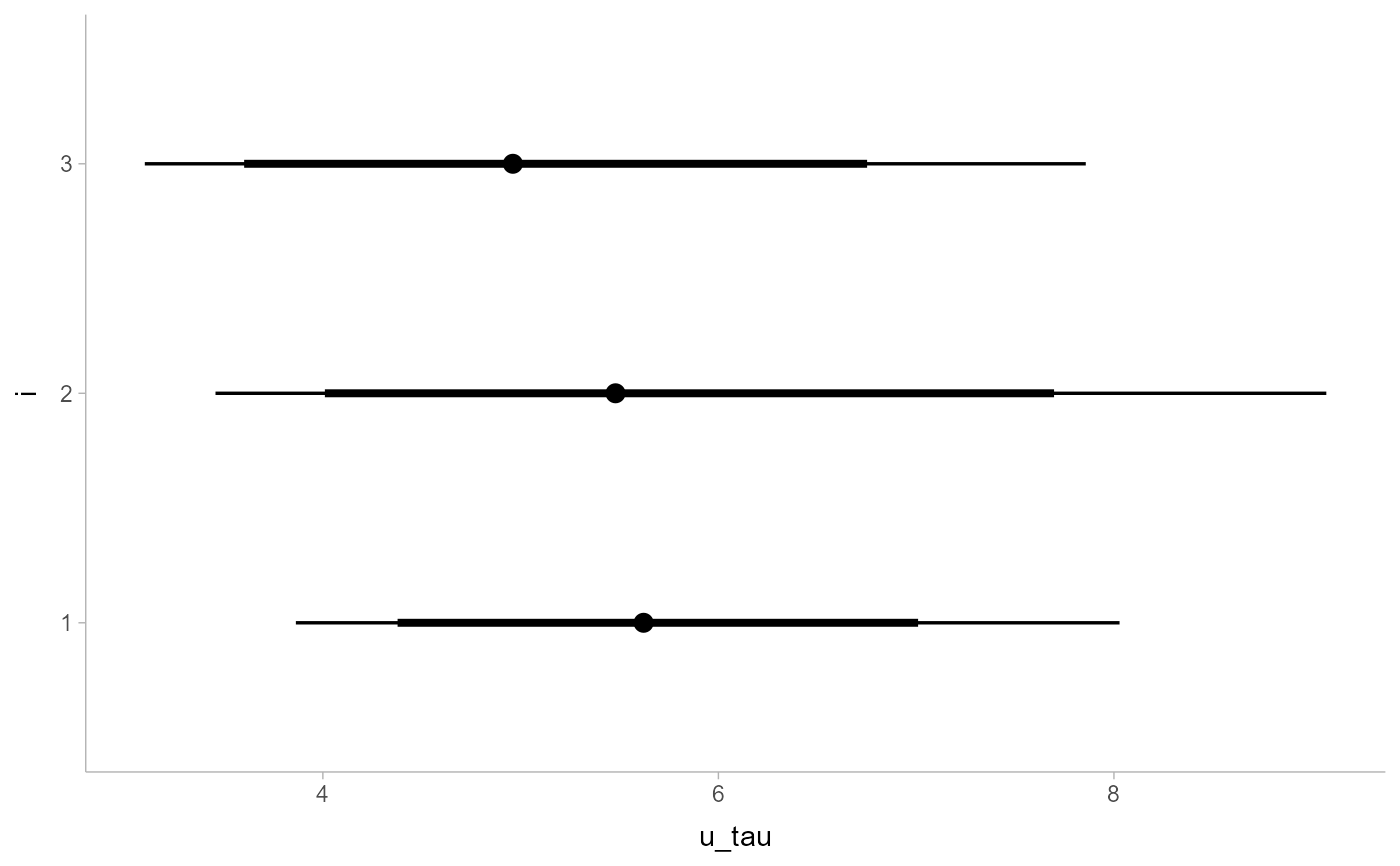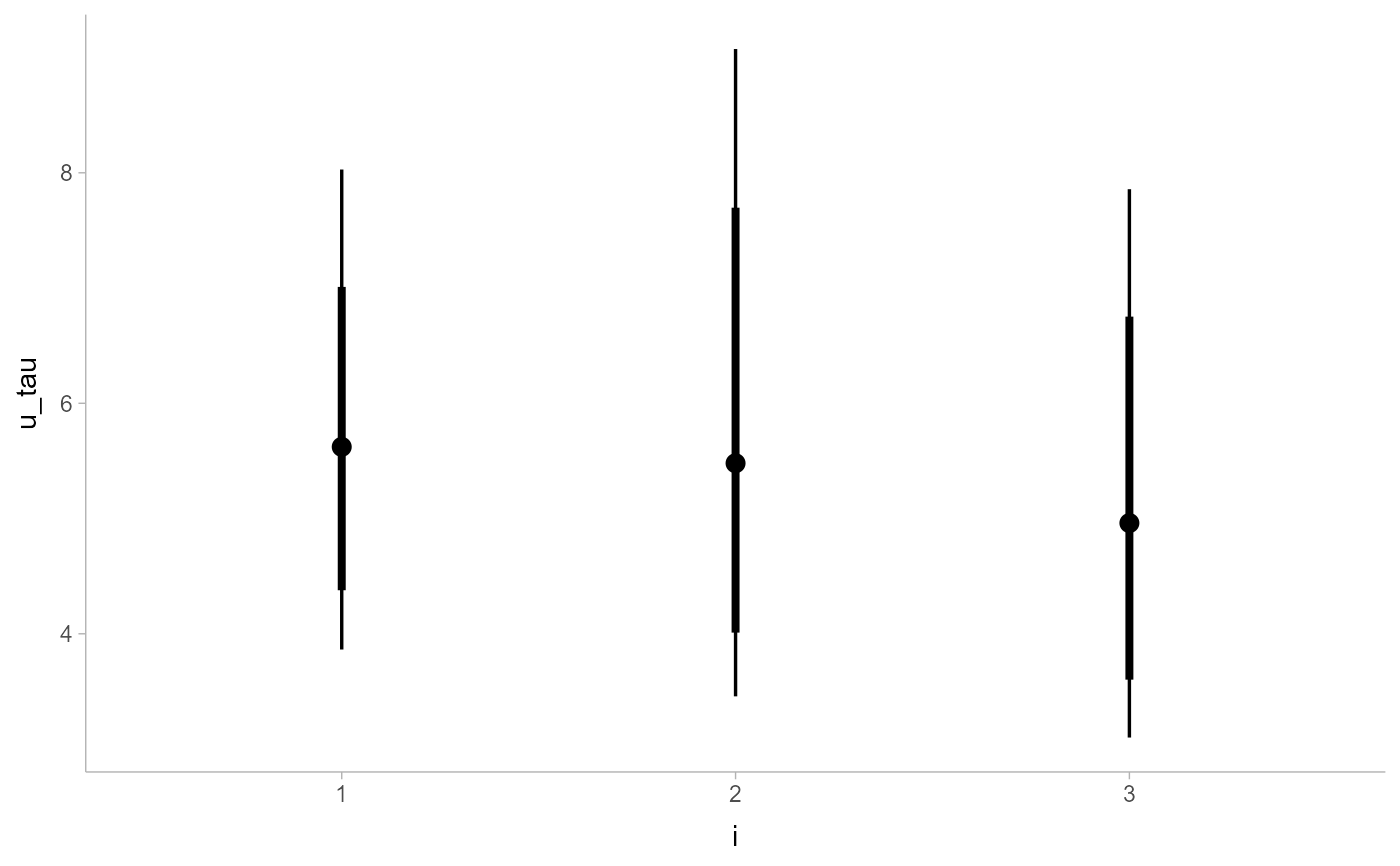Shortcut version of geom_slabinterval() for creating point + multiple-interval plots.

Roughly equivalent to:

geom_slabinterval(

aes(datatype = "interval", side = "both"),

show_slab = FALSE,

show.legend = c(size = FALSE)

)

geom_pointinterval(
mapping = NULL,
data = NULL,
stat = "identity",
position = "identity",
...,
orientation = NA,
interval_size_domain = c(1, 6),
interval_size_range = c(0.6, 1.4),
fatten_point = 1.8,
na.rm = FALSE,
show.legend = c(size = FALSE),
inherit.aes = TRUE
)

## Arguments

mapping

Set of aesthetic mappings created by aes(). If specified and inherit.aes = TRUE (the default), it is combined with the default mapping at the top level of the plot. You must supply mapping if there is no plot mapping.

data

The data to be displayed in this layer. There are three options:

If NULL, the default, the data is inherited from the plot data as specified in the call to ggplot().

A data.frame, or other object, will override the plot data. All objects will be fortified to produce a data frame. See fortify() for which variables will be created.

A function will be called with a single argument, the plot data. The return value must be a data.frame, and will be used as the layer data. A function can be created from a formula (e.g. ~ head(.x, 10)).

stat

The statistical transformation to use on the data for this layer, either as a ggproto Geom subclass or as a string naming the stat stripped of the stat_ prefix (e.g. "count" rather than "stat_count")

position

Position adjustment, either as a string, or the result of a call to a position adjustment function. Setting this equal to "dodge" (position_dodge()) or "dodgejust" (position_dodgejust()) can be useful if you have overlapping geometries.

...

Other arguments passed to layer(). These are often aesthetics, used to set an aesthetic to a fixed value, like colour = "red" or linewidth = 3 (see Aesthetics, below). They may also be parameters to the paired geom/stat.

orientation

Whether this geom is drawn horizontally or vertically. One of:

• NA (default): automatically detect the orientation based on how the aesthetics are assigned. Automatic detection works most of the time.

• "horizontal" (or "y"): draw horizontally, using the y aesthetic to identify different groups. For each group, uses the x, xmin, xmax, and thickness aesthetics to draw points, intervals, and slabs.

• "vertical" (or "x"): draw vertically, using the x aesthetic to identify different groups. For each group, uses the y, ymin, ymax, and thickness aesthetics to draw points, intervals, and slabs.

For compatibility with the base ggplot naming scheme for orientation, "x" can be used as an alias for "vertical" and "y" as an alias for "horizontal" (ggdist had an orientation parameter before base ggplot did, hence the discrepancy).

interval_size_domain

A length-2 numeric vector giving the minimum and maximum of the values of the size and linewidth aesthetics that will be translated into actual sizes for intervals drawn according to interval_size_range (see the documentation for that argument.)

interval_size_range

A length-2 numeric vector. This geom scales the raw size aesthetic values when drawing interval and point sizes, as they tend to be too thick when using the default settings of scale_size_continuous(), which give sizes with a range of c(1, 6). The interval_size_domain value indicates the input domain of raw size values (typically this should be equal to the value of the range argument of the scale_size_continuous() function), and interval_size_range indicates the desired output range of the size values (the min and max of the actual sizes used to draw intervals). Most of the time it is not recommended to change the value of this argument, as it may result in strange scaling of legends; this argument is a holdover from earlier versions that did not have size aesthetics targeting the point and interval separately. If you want to adjust the size of the interval or points separately, you can also use the linewidth or point_size aesthetics; see scales.

fatten_point

A multiplicative factor used to adjust the size of the point relative to the size of the thickest interval line. If you wish to specify point sizes directly, you can also use the point_size aesthetic and scale_point_size_continuous() or scale_point_size_discrete(); sizes specified with that aesthetic will not be adjusted using fatten_point.

na.rm

If FALSE, the default, missing values are removed with a warning. If TRUE, missing values are silently removed.

show.legend

Should this layer be included in the legends? Default is c(size = FALSE), unlike most geoms, to match its common use cases. FALSE hides all legends, TRUE shows all legends, and NA shows only those that are mapped (the default for most geoms).

inherit.aes

If FALSE, overrides the default aesthetics, rather than combining with them. This is most useful for helper functions that define both data and aesthetics and shouldn't inherit behaviour from the default plot specification, e.g. borders().

## Value

A ggplot2::Geom representing a point + multiple-interval geometry which can be added to a ggplot() object.

## Details

This geom wraps geom_slabinterval() with defaults designed to produce point + multiple-interval plots. Default aesthetic mappings are applied if the .width column is present in the input data (e.g., as generated by the point_interval() family of functions), making this geom often more convenient than vanilla ggplot2 geometries when used with functions like median_qi(), mean_qi(), mode_hdi(), etc.

Specifically, if .width is present in the input, geom_pointinterval() acts as if its default aesthetics are aes(size = -.width)

## Aesthetics

The slab+interval stats and geoms have a wide variety of aesthetics that control the appearance of their three sub-geometries: the slab, the point, and the interval.

Positional aesthetics

• x: x position of the geometry

• y: y position of the geometry

Interval-specific aesthetics

• xmin: Left end of the interval sub-geometry (if orientation = "horizontal").

• xmax: Right end of the interval sub-geometry (if orientation = "horizontal").

• ymin: Lower end of the interval sub-geometry (if orientation = "vertical").

• ymax: Upper end of the interval sub-geometry (if orientation = "vertical").

Point-specific aesthetics

• shape: Shape type used to draw the point sub-geometry.

Color aesthetics

• colour: (or color) The color of the interval and point sub-geometries. Use the slab_color, interval_color, or point_color aesthetics (below) to set sub-geometry colors separately.

• fill: The fill color of the slab and point sub-geometries. Use the slab_fill or point_fill aesthetics (below) to set sub-geometry colors separately.

• alpha: The opacity of the slab, interval, and point sub-geometries. Use the slab_alpha, interval_alpha, or point_alpha aesthetics (below) to set sub-geometry colors separately.

• colour_ramp: (or color_ramp) A secondary scale that modifies the color scale to "ramp" to another color. See scale_colour_ramp() for examples.

• fill_ramp: A secondary scale that modifies the fill scale to "ramp" to another color. See scale_fill_ramp() for examples.

Line aesthetics

• linewidth: Width of the line used to draw the interval (except with geom_slab(): then it is the width of the slab). With composite geometries including an interval and slab, use slab_linewidth to set the line width of the slab (see below). For interval, raw linewidth values are transformed according to the interval_size_domain and interval_size_range parameters of the geom (see above).

• size: Determines the size of the point. If linewidth is not provided, size will also determines the width of the line used to draw the interval (this allows line width and point size to be modified together by setting only size and not linewidth). Raw size values are transformed according to the interval_size_domain, interval_size_range, and fatten_point parameters of the geom (see above). Use the point_size aesthetic (below) to set sub-geometry size directly without applying the effects of interval_size_domain, interval_size_range, and fatten_point.

• stroke: Width of the outline around the point sub-geometry.

• linetype: Type of line (e.g., "solid", "dashed", etc) used to draw the interval and the outline of the slab (if it is visible). Use the slab_linetype or interval_linetype aesthetics (below) to set sub-geometry line types separately.

Interval-specific color/line override aesthetics

• interval_colour: (or interval_color) Override for colour/color: the color of the interval.

• interval_alpha: Override for alpha: the opacity of the interval.

• interval_linetype: Override for linetype: the line type of the interval.

Point-specific color/line override aesthetics

• point_fill: Override for fill: the fill color of the point.

• point_colour: (or point_color) Override for colour/color: the outline color of the point.

• point_alpha: Override for alpha: the opacity of the point.

• point_size: Override for size: the size of the point.

Deprecated aesthetics

• interval_size: Use interval_linewidth.

Other aesthetics (these work as in standard geoms)

• width

• height

• group

See examples of some of these aesthetics in action in vignette("slabinterval"). Learn more about the sub-geom override aesthetics (like interval_color) in the scales documentation. Learn more about basic ggplot aesthetics in vignette("ggplot2-specs").

See stat_pointinterval() for the stat version, intended for use on sample data or analytical distributions. See geom_slabinterval() for the geometry this shortcut is based on.

Other slabinterval geoms: geom_interval(), geom_slab(), geom_spike()

## Examples


library(dplyr)
library(ggplot2)

data(RankCorr_u_tau, package = "ggdist")

# orientation is detected automatically based on
# use of xmin/xmax or ymin/ymax

RankCorr_u_tau %>%
group_by(i) %>%
median_qi(.width = c(.8, .95)) %>%
ggplot(aes(y = i, x = u_tau, xmin = .lower, xmax = .upper)) +
geom_pointinterval()RankCorr_u_tau %>%
group_by(i) %>%
median_qi(.width = c(.8, .95)) %>%
ggplot(aes(x = i, y = u_tau, ymin = .lower, ymax = .upper)) +
geom_pointinterval()